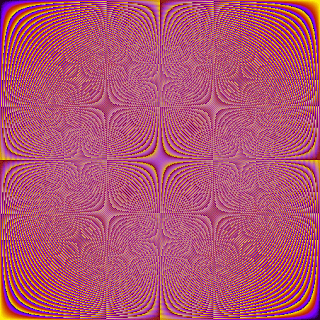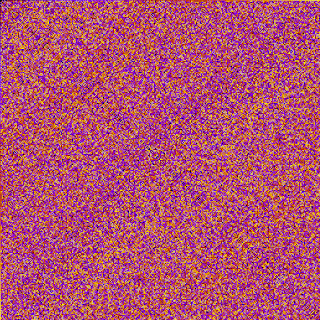## Tuesday, January 13, 2009

### Algebraic Group Visualization

There are some quite interesting visualizations of groups at a blog called Alice and Bob in Cryptoland. What the author has done is to add colours to the group table for several mappings so as to give you some idea about the inherent structure of the mapping.

The diagram below represents multiplication modulo 509. Each number 1, 2, ..., 507, 508 is assigned a colour. Then the multiplication table is formed from a 509 x 509 table where the entry in the i-th row and j-th column is (i*j) mod 509, then its value substituted for its defined colour. A definite quadrant structure is present.A second visualation is given for an elliptic curve group defined over GF(503). This group contains 503 elements, which are assigned colours and then represented in a 503 x 503 addition map (elliptic curves are additive rather than multiplicative as the group above).The result is far more random-looking mapping, which you would expect since elliptic curves exhibit less inherent structure than numeric groups. You can obtain the code for these graphs from the orginal post.

#### 1 comment:Anonymous said...

Emensuits give big discount in all sits products such as [mens suit to check more visit at emensuits.com